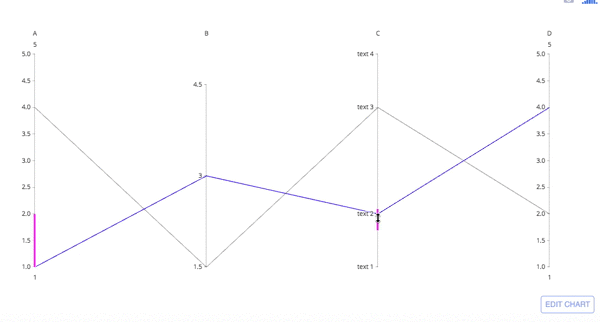Show Sidebar Hide Sidebar# Parallel Coordinates Plot in R

How to create parallel coordinates plots in R with Plotly.

### New to Plotly?

Plotly's R library is free and open source!
You can set up Plotly to work in online or offline mode.
We also have a quick-reference cheatsheet (new!) to help you get started!

### Version Check

Version 4 of Plotly's R package is now available!
Check out this post for more information on breaking changes and new features available in this version.

library(plotly)
packageVersion('plotly')

##  '4.5.6.9000'


library(plotly)

p <- plot_ly(type = 'parcoords', line = list(color = 'blue'),
dimensions = list(
list(range = c(1,5),
constraintrange = c(1,2),
label = 'A', values = c(1,4)),
list(range = c(1,5),
tickvals = c(1.5,3,4.5),
label = 'B', values = c(3,1.5)),
list(range = c(1,5),
tickvals = c(1,2,4,5),
label = 'C', values = c(2,4),
ticktext = c('text 1', 'text 2', 'text 3', 'text 4')),
list(range = c(1,5),
label = 'D', values = c(4,2))
)
)

# Set up API credentials: https://plot.ly/r/getting-started


Parallel coordinates are richly interactive by default. Drag the lines along the axes to filter regions and drag the axis names across the plot to rearrange variables:### Basic Parallel Cordinates Plot

library(plotly)

p <- df %>%
plot_ly(type = 'parcoords',
line = list(color = ~species_id,
colorscale = list(c(0,'red'),c(0.5,'green'),c(1,'blue'))),
dimensions = list(
list(range = c(2,4.5),
label = 'Sepal Width', values = ~sepal_width),
list(range = c(4,8),
constraintrange = c(5,6),
label = 'Sepal Length', values = ~sepal_length),
list(range = c(0,2.5),
label = 'Petal Width', values = ~petal_width),
list(range = c(1,7),
label = 'Petal Length', values = ~petal_length)
)
)

# Set up API credentials: https://plot.ly/r/getting-started


library(plotly)

p <- df %>%
plot_ly(width = 1000, height = 600) %>%
line = list(color = ~colorVal,
colorscale = 'Jet',
showscale = TRUE,
reversescale = TRUE,
cmin = -4000,
cmax = -100),
dimensions = list(
list(range = c(~min(blockHeight),~max(blockHeight)),
constraintrange = c(100000,150000),
label = 'Block Height', values = ~blockHeight),
list(range = c(~min(blockWidth),~max(blockWidth)),
label = 'Block Width', values = ~blockWidth),
list(tickvals = c(0,0.5,1,2,3),
ticktext = c('A','AB','B','Y','Z'),
label = 'Cyclinder Material', values = ~cycMaterial),
list(range = c(-1,4),
tickvals = c(0,1,2,3),
label = 'Block Material', values = ~blockMaterial),
list(range = c(~min(totalWeight),~max(totalWeight)),
visible = TRUE,
label = 'Total Weight', values = ~totalWeight),
list(range = c(~min(assemblyPW),~max(assemblyPW)),
label = 'Assembly Penalty Weight', values = ~assemblyPW),
list(range = c(~min(HstW),~max(HstW)),
label = 'Height st Width', values = ~HstW),
list(range = c(~min(minHW),~max(minHW)),
label = 'Min Height Width', values = ~minHW),
list(range = c(~min(minWD),~max(minWD)),
label = 'Min Width Diameter', values = ~minWD),
list(range = c(~min(rfBlock),~max(rfBlock)),
label = 'RF Block', values = ~rfBlock)
)
)